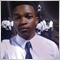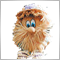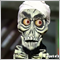# iCustom1238

Hello everyone.
For a while now, I have been trying to learn how to use iCustom. I have limited sources and I get stuck quite often.
I really need a workaround on how to let my EA know when a buffer plots an arrow, or detect the colour change.
A sample code will be highly appreciated.
Save a brother😔Moderator
216400

Nelson Wanyama :
Hello everyone.
For a while now, I have been trying to learn how to use iCustom . I have limited sources and I get stuck quite often.
I really need a workaround on how to let my EA know when a buffer plots an arrow, or detect the colour change.
A sample code will be highly appreciated.
Save a brother😔

It's simple. An example based on the Two Rivers Alert custom indicator. The indicator has two buffers ("Up" and "Down") - when an signal appears in the buffer, an arrow appears. How to check: is there a signal or not? Very simple:

• if there is a signal "arrow" - then the price is in the indicator buffer
• if there is no signal, then the value “0.0” is in the indicator buffer. The value "0.0" is used in this indicator, for this you need to look at the indicator code:
```   for(int i=limit; i<rates_total; i++)
{
//Если есть сигнал к покупке(Свеча пересекла полностью) - поставим синюю стрелку вверх
if(open[i]<=iMALowBuffer[i] && close[i]>iMAHighBuffer[i] && close[i]>open[i])
{
UpBuffer[i]=high[i];//запомним цену синей стрелки
if(prev_calculated!=0)
{
{
}
}
}
else
UpBuffer[i]=0.0;
//Если есть сигнал к продаже - поставим красную стрелку вниз
if(open[i]>=iMAHighBuffer[i] && close[i]<iMALowBuffer[i] && close[i]<open[i])
{
DownBuffer[i]=low[i];//запомним цену красной стрелки
if(prev_calculated!=0)
{
{
}
}
}
else
DownBuffer[i]=0.0;
}
//--- return value of prev_calculated for next call
return(rates_total);
}```

```//+------------------------------------------------------------------+
//|                                           http://wmua.ru/slesar/ |
//+------------------------------------------------------------------+
#property version   "1.00"
//--- input parameters
input int                  Inp_MA_ma_period     = 20;           // MA's: averaging period
input int                  Inp_MA_ma_shift      = 0;            // MA's: horizontal shift
input ENUM_MA_METHOD       Inp_MA_ma_method     = MODE_LWMA;    // MA's: smoothing type
//---
int    handle_iCustom;                       // variable for storing the handle of the iCustom indicator
//+------------------------------------------------------------------+
//| Expert initialization function                                   |
//+------------------------------------------------------------------+
int OnInit()
{
//--- create handle of the indicator iCustom
//--- if the handle is not created
if(handle_iCustom==INVALID_HANDLE)
{
//--- tell about the failure and output the error code
PrintFormat("Failed to create handle of the iCustom indicator for the symbol %s/%s, error code %d",
Symbol(),
EnumToString(Period()),
GetLastError());
//--- the indicator is stopped early
return(INIT_FAILED);
}
//---
return(INIT_SUCCEEDED);
}
//+------------------------------------------------------------------+
//| Expert deinitialization function                                 |
//+------------------------------------------------------------------+
void OnDeinit(const int reason)
{
//---

}
//+------------------------------------------------------------------+
//| Expert tick function                                             |
//+------------------------------------------------------------------+
void OnTick()
{
//---
double up[],down[];
ArraySetAsSeries(up,true);
ArraySetAsSeries(down,true);
int start_pos=0,count=3;
if(!iGetArray(handle_iCustom,0,start_pos,count,up) ||
!iGetArray(handle_iCustom,1,start_pos,count,down))
{
return;
}
//---
if(up!=0.0)
{
//--- signal "Up" in bar #0
int d=0;
}
if(up!=0.0)
{
//--- signal "Up" in bar #1
int d=0;
}
if(down!=0.0)
{
//--- signal "Down" in bar #0
int d=0;
}
if(down!=0.0)
{
//--- signal "Down" in bar #1
int d=0;
}
}
//+------------------------------------------------------------------+
//| Get value of buffers                                             |
//+------------------------------------------------------------------+
bool iGetArray(const int handle,const int buffer,const int start_pos,
const int count,double &arr_buffer[])
{
bool result=true;
if(!ArrayIsDynamic(arr_buffer))
{
PrintFormat("ERROR! EA: %s, FUNCTION: %s, this a no dynamic array!",__FILE__,__FUNCTION__);
return(false);
}
ArrayFree(arr_buffer);
//--- reset error code
ResetLastError();
//--- fill a part of the iBands array with values from the indicator buffer
int copied=CopyBuffer(handle,buffer,start_pos,count,arr_buffer);
if(copied!=count)
{
//--- if the copying fails, tell the error code
PrintFormat("ERROR! EA: %s, FUNCTION: %s, amount to copy: %d, copied: %d, error code %d",
__FILE__,__FUNCTION__,count,copied,GetLastError());
//--- quit with zero result - it means that the indicator is considered as not calculated
return(false);
}
return(result);
}
//+------------------------------------------------------------------+
```

Files:1238

It's simple. An example based on the Two Rivers Alert custom indicator. The indicator has two buffers ("Up" and "Down") - when an signal appears in the buffer, an arrow appears. How to check: is there a signal or not? Very simple:

• if there is a signal "arrow" - then the price is in the indicator buffer
• if there is no signal, then the value “0.0” is in the indicator buffer. The value "0.0" is used in this indicator, for this you need to look at the indicator code:

Thank you very much for the timely response.4425

For Indicators I don't have source code for
I like to open my MT terminal ctrl+D for data window then check how many buffers I am dealing with then identify my budget of interest.

Then I look at the input parameters for the indicator, and I note all the parameter settings and their values, then I write my iCustom function

NB: Will post Code Here I'm using Mobile and can't insert it using the Code tool.

MT4
double indicatorValue = iCustom(m_symbol, timeframe, path,/* [Parameters noted above separate by comma (,) ]*/,buffer_number,shift);

MT5
Oninit
Int IndiHandle = iCustom(m_symbol,timeframe,path,/* [Parameters noted above separate by comma (,) ]*/);

OnTick
int copy = CopyBuffer( IndiHandle ,buffer_number,0,count, indicatorValueArray);

Though I hv never worked with MT5 lol1238

Jefferson Metha:
For Indicators I don't have source code for
I like to open my MT terminal ctrl+D for data window then check how many buffers I am dealing with then identify my budget of interest.

Then I look at the input parameters for the indicator, and I note all the parameter settings and their values, then I write my iCustom function

NB: Will post Code Here I'm using Mobile and can't insert it using the Code tool.

MT4
double indicatorValue = iCustom(m_symbol, timeframe, path,/* [Parameters noted above separate by comma (,) ]*/,buffer_number,shift);

MT5
Oninit
Int IndiHandle = iCustom(m_symbol,timeframe,path,/* [Parameters noted above separate by comma (,) ]*/);

OnTick
int copy = CopyBuffer( IndiHandle ,buffer_number,0,count, indicatorValueArray);

Though I hv never worked with MT5 lol

Thank you. I work with mt5 only. I found a work around for working with iCustom, thanks to Vlamidir Kaputov. Been practicing with different Indicators and using comments to figure  out the output of each buffer. I'm learning great!